# Checking Proof of Theorem 6.2.8 Part (ii)

• MHB
• Math Amateur

#### Math Amateur

Gold Member
MHB
I have completed a formal proof of D&K Theorem 6.2.8 Part (ii) ... but I am unsure of whether the proof is correct ... so I would be most grateful if someone could check the proof and point out any errors or shortcomings ...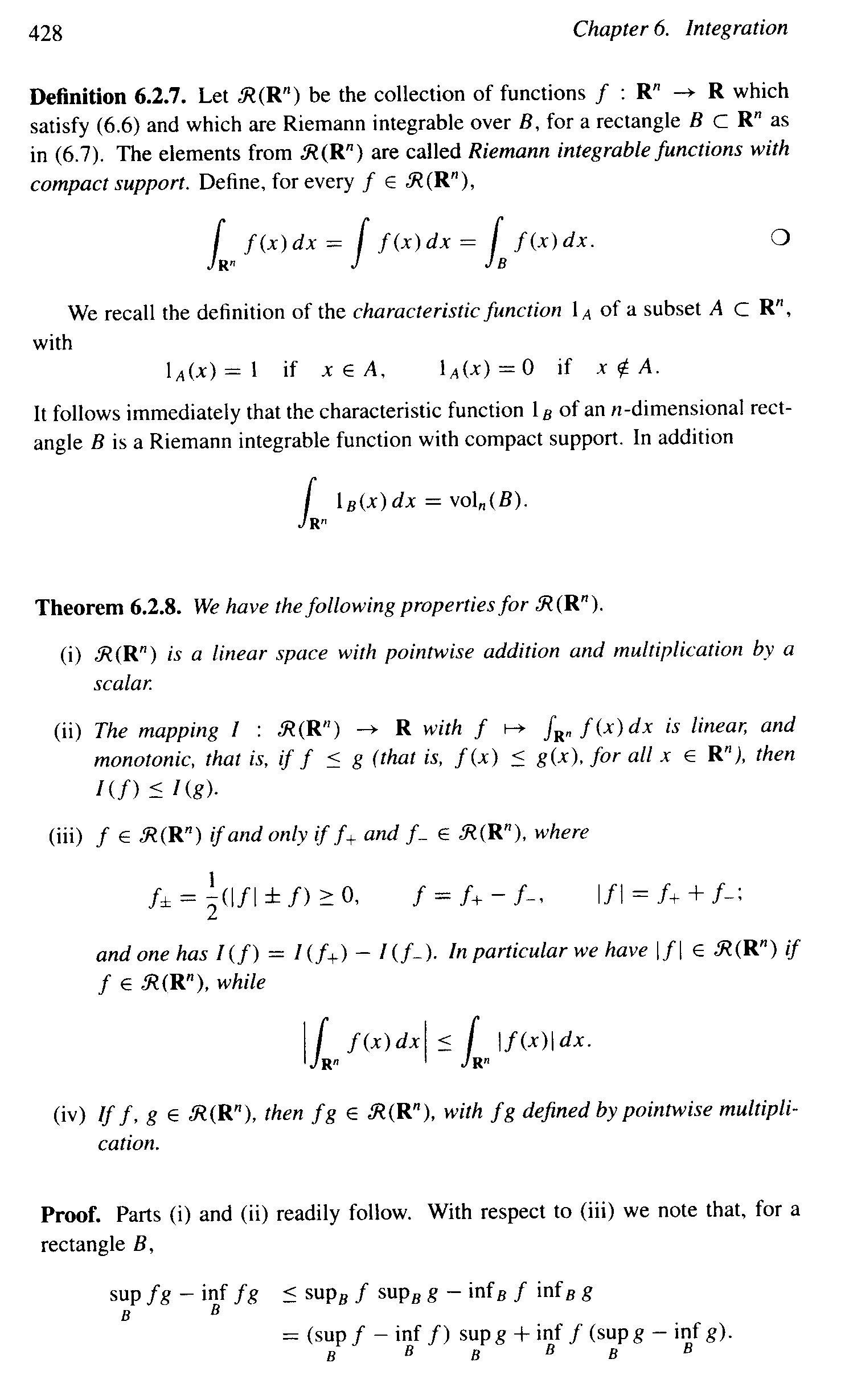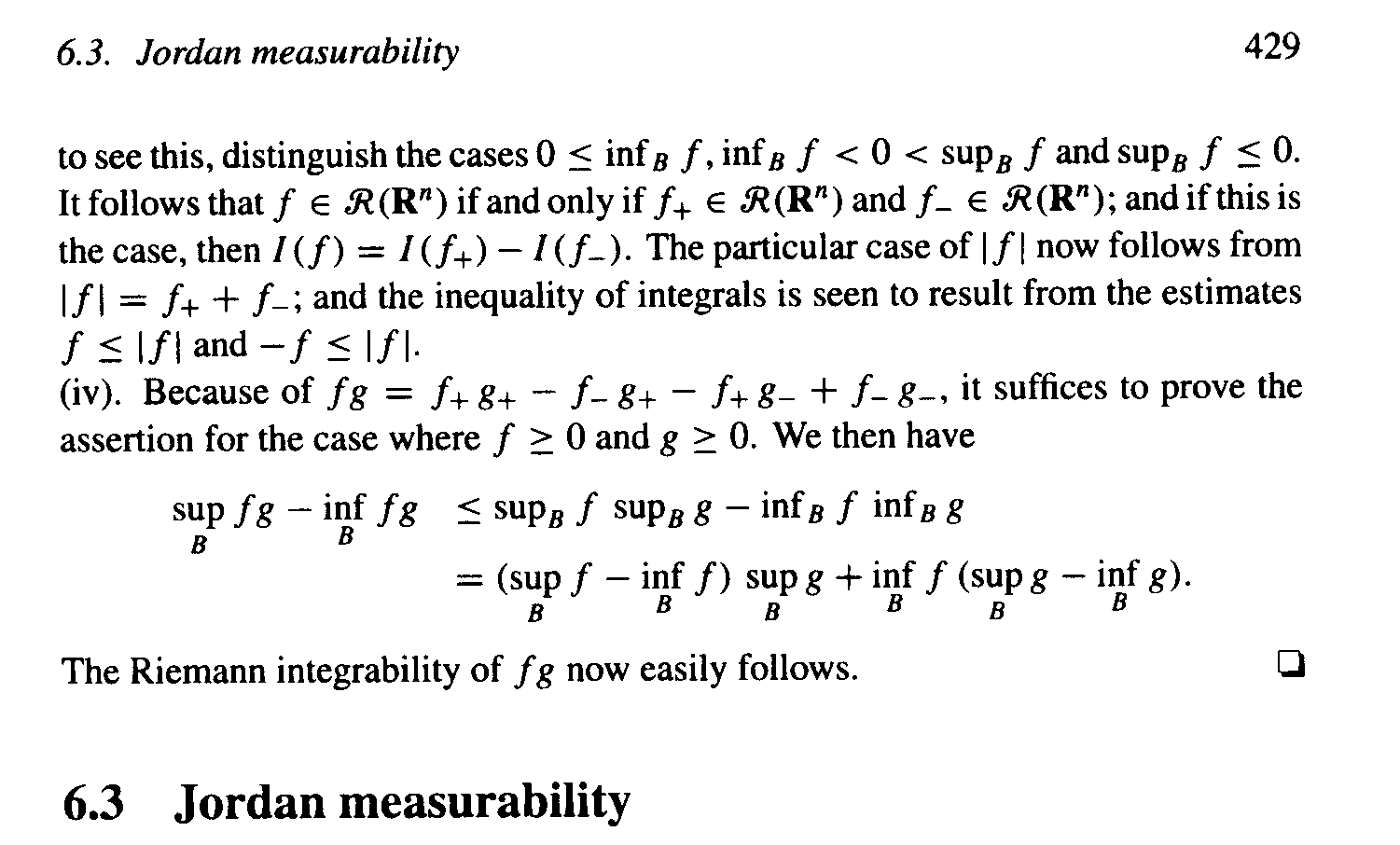Attempted Proof of Theorem 6.2.8 Part (ii)

We have to show:

$$\displaystyle f \leq g \Longrightarrow I(f) \leq I(g)$$

where

$$\displaystyle I(f) = \int_{ \mathbb{R^n} } f(x) dx = \int_{B} f(x) dx$$

and

$$\displaystyle I(g) = \int_{ \mathbb{R^n} } g(x) dx = \int_{B} g(x) dx$$

Proof:

Given a partition $$\displaystyle \mathscr{B} \text{ of } B$$ we have

$$\displaystyle \overline{S} (f, \mathscr{B} ) \ = \ \sum_{j \in J} \ \sup_{ x \in B_j} \ f(x) dx \text{ vol}_n (B_j)$$

and

$$\displaystyle \overline{S} (g, \mathscr{B} ) \ = \ \sum_{j \in J} \ \sup_{ x \in B_j} \ g(x) dx \text{ vol}_n (B_j)$$

Now, since $$\displaystyle f(x) \leq g(x) \ \ for all x \in B$$ we have

$$\displaystyle \overline{S} (f, \mathscr{B} ) \ \leq \ \ \overline{S} (g, \mathscr{B} )$$

it follows that:

$$\displaystyle \overline{ \int_{B} } f(x) dx = \text{ inf} \ \{ \overline{S} (f, \mathscr{B} ) \ \ | \ \ \mathscr{B} \text{ is a partition of } B \} \ \ \leq \ \ \text{ inf} \ \{ \overline{S} (g, \mathscr{B} ) \ \ | \ \ \mathscr{B} \text{ is a partition of } B \} \ = \ \overline{ \int_{B} } g(x) dx$$ ... ... ... ... ... (1)

Similarly ... ... $$\displaystyle \underline{ \int_{B} } f(x) dx \leq \underline{ \int_{B} } g(x) dx$$ ... ... ... ... ... (2)

(1), (2) $$\displaystyle \Longrightarrow \int_{B} f(x) dx \leq \int_{B} g(x) dx$$ ... ...

... ... that is ... ...

$$\displaystyle I(f) \leq I(g)$$

Could someone please check my proof for correctness ... and point out any errors, shortcomings and areas needing improvement ...

Peter

NOTE:

It may help readers of the above post to have access to D&K Section 6.2 so as to be able to check the way the authors present the theory and also to check notation so I am providing a scan of section 6.2 as it reads before Theorem 6.2.8 ... see below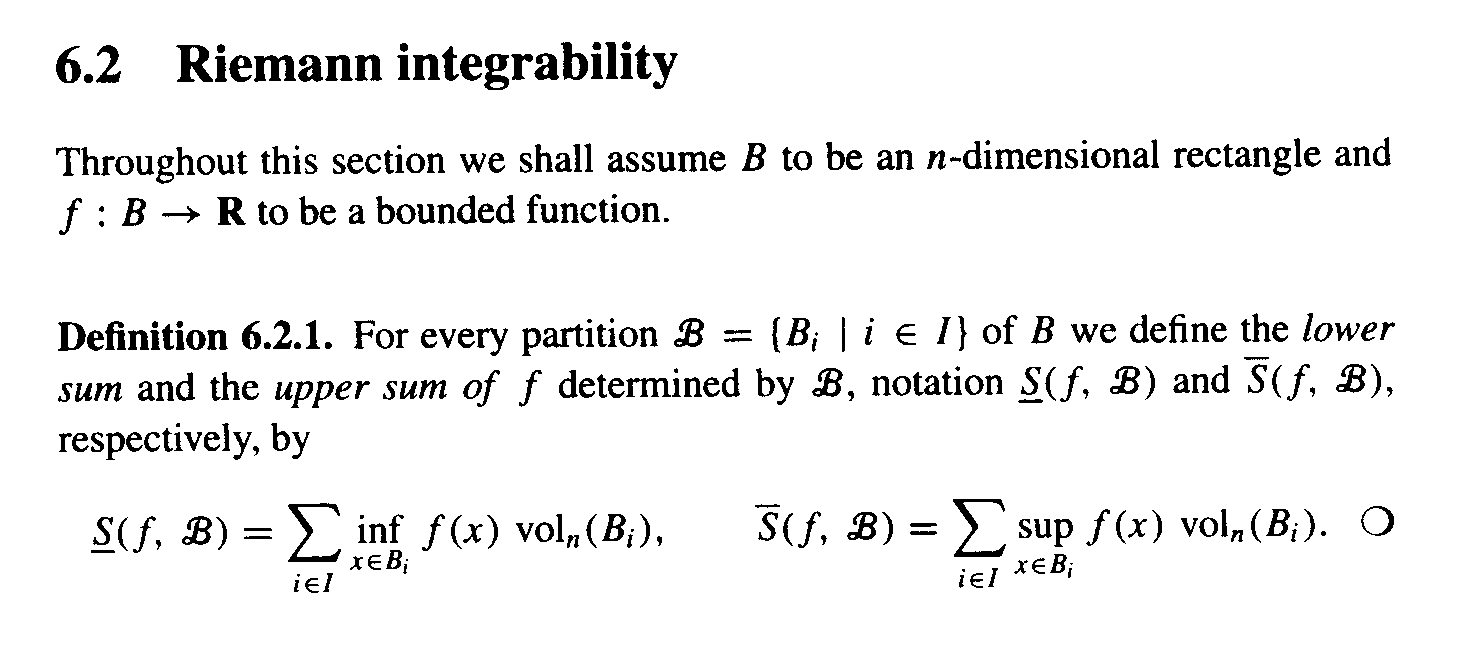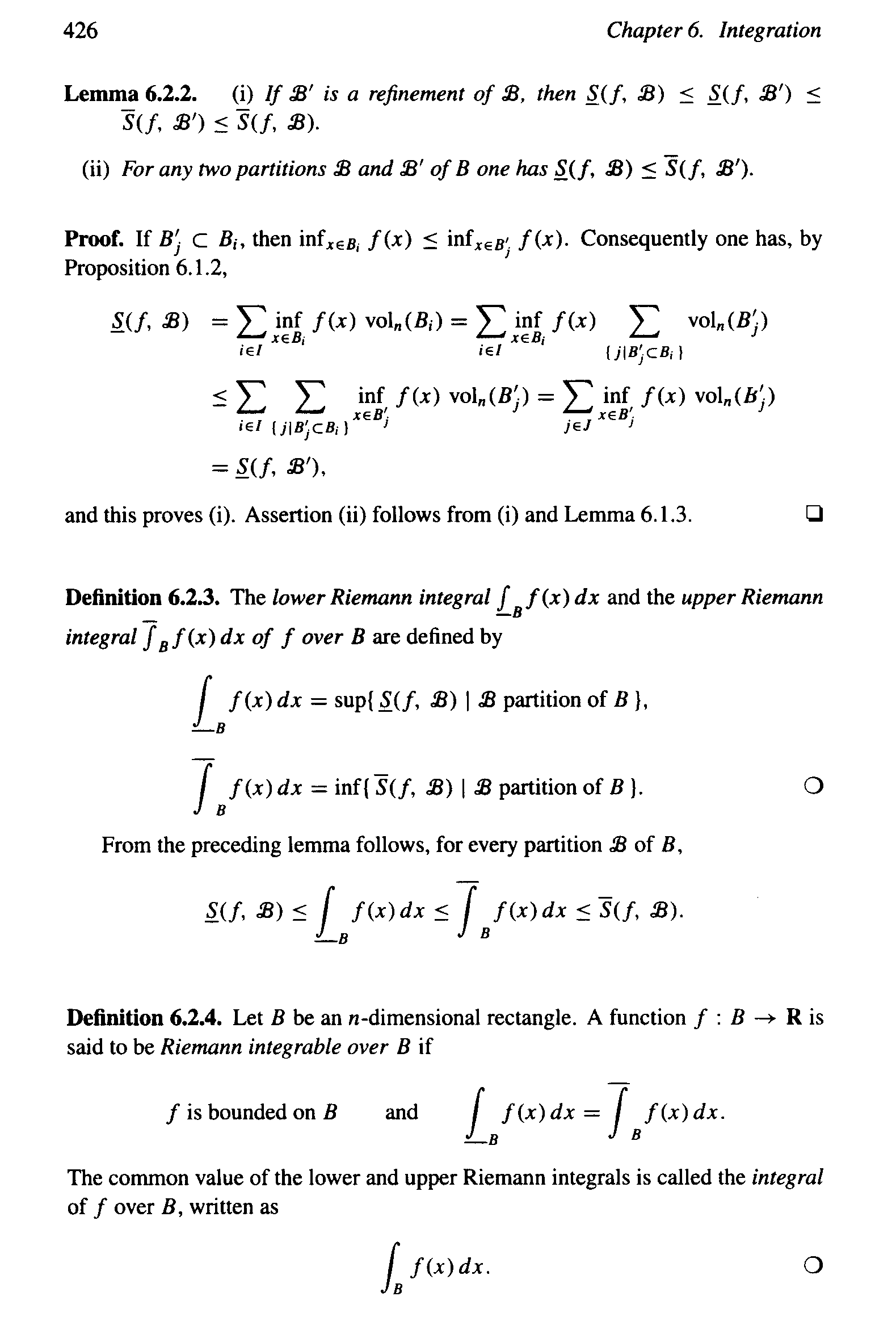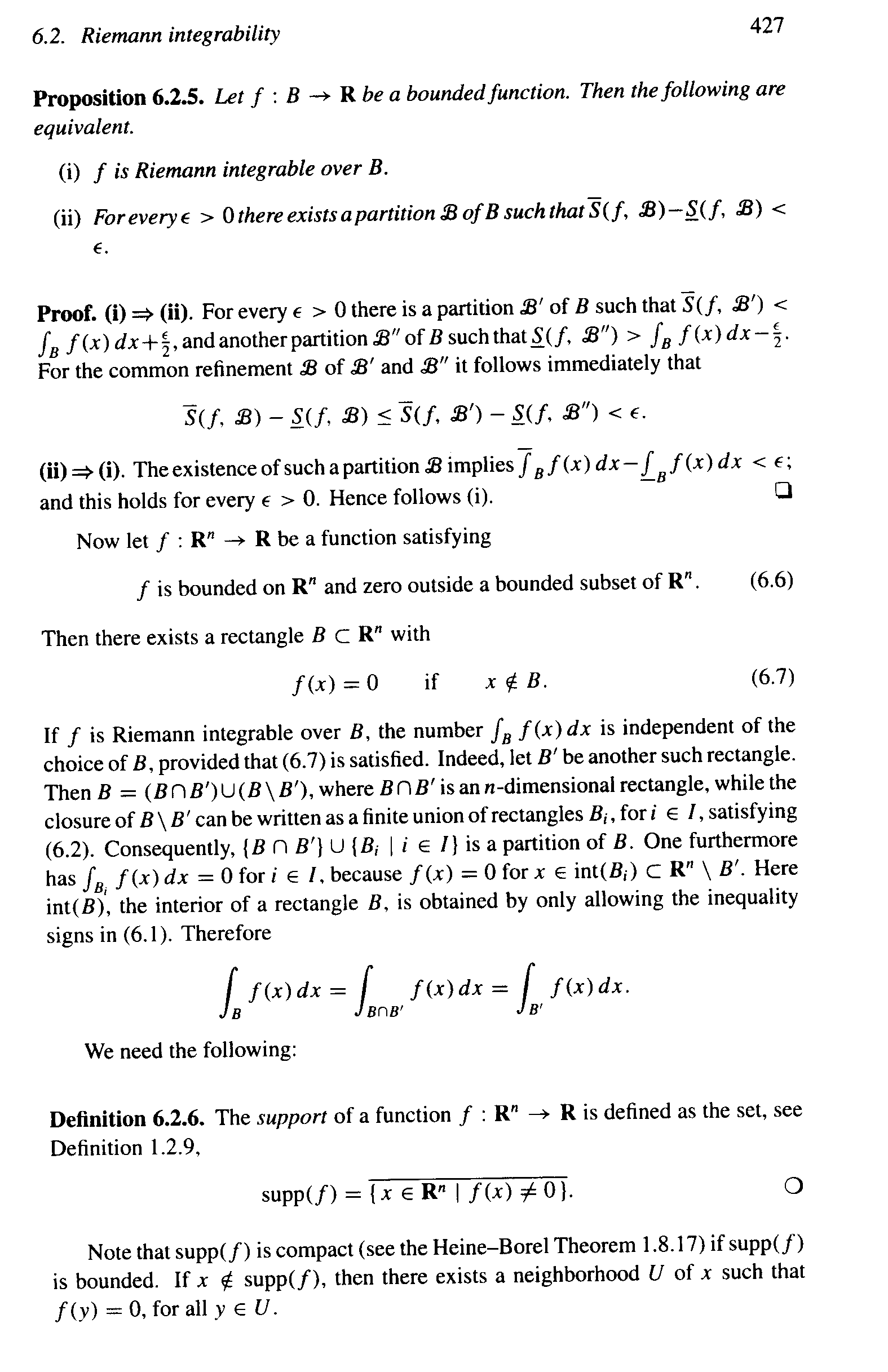Hope that helps ...

Peter

Last edited:
Hi Peter

Proof looks good. Two small comments:
1. It is true that since both $f$ and $g$ are bounded with compact support, it is possible to select a single rectangle $B$ for which $f(x) = g(x) = 0$ for $x\notin B$. I think to improve the rigor of your proof, it is worth saying something like this at the beginning of the argument because, technically speaking, $f,g\in\mathcal{R}(\mathbb{R}^{n})$ imply there are rectangles $B_{f}$ and $B_{g}$ specific to $f$ and $g$, respectively. Again, your proof is rigorous enough in my eyes, but I do think emphasizing this point would improve the precision a bit.
2. When you say "Similarly ... ... $$\displaystyle \underline{ \int_{B} } f(x) dx \leq \underline{ \int_{B} } g(x) dx$$ ...," you are correct that an analogous argument would establish the inequality for the lower Riemann integrals. However, you do not need to actually mention this. Can you see why? By assuming $f,g\in\mathcal{R}(\mathbb{R}^{n})$, what do we know about the upper and lower Riemann integrals?
Hopefully these comments help. Let me know if the question I've posed in part 2 above is unclear.